Next: Real-Time Symmetry Transform Up: Perceptual Contrast, Symmetry and Previous: Edge Data Enhancement

# Multi-Scale Analysis

We note at this point the significance of scale and the scalability of certain types of edge detection. Since structures and objects in an input image can have different sizes and resolutions, most spatial operators (for edge extraction or further processing) will require scalability.

As demonstrated previously, the scalability of the Deriche edge detection operation makes it more flexible than the highly local Sobel operator. The objects in an input image and the image itself can have different sizes and resolutions so a scalable operator is necessary to search for edges at different scales. Kelly and Levine  have approached edge detection by applying a Deriche-like operator over many scales. By changing the operator's size, several edge maps (one for each scale) are obtained and subsequently processed in parallel. Elder  proposes yet another technique wherein a single edge map is produced by computing the optimal scale of edge detection for each position of the input image and then performing scale-adaptive edge detection. In other words, the scale of the operator is varied appropriately at each position in the image.

However, scalable edge detection is too complex to be performed rapidly. Additionally, subsequent operators (i.e., for computing symmetric enclosure) might also be computationally inefficient when they are scaled. One solution'' to this issue is to scale the input image instead of enlarging the operators themselves. Thus, a pyramid is formed from a single input image by reducing it by various scale factors. The resulting set of scaled images can then be processed by an operator of fixed size (such as the Sobel operator).

Given an image I(i,j) of dimensions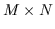, a scaled version,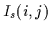, of the image at a scale factor of s can be obtained with dimensions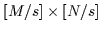by either subsampling the image or subaveraging it. The following describes the operations required for scaling by an integer factor s (although non-integer scaling is possible as well).

Subsampling
Subsampling involves selecting a sample from each neighbourhood of pixels of size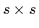. Each sample will be used as a pixel value in the scaled image. Subsampling assumes that the sample appropriately represents its neighbourhood and that the image is not dominated by high-frequency data. This process only requirescomputations.
Subaveraging
Subaveraging is similar to subsampling except that the sample taken from each neighbourhood of pixels has an intensity that is the average intensity in the neighbourhood. Thus, the original image undergoes low-pass filtering before being sampled so that the scaled image approximates the original optimally even if it has high frequency data. To avoid possible errors when scaling high frequency components in the image, we choose to implement subaveraging instead of subsampling. This process requirescomputations.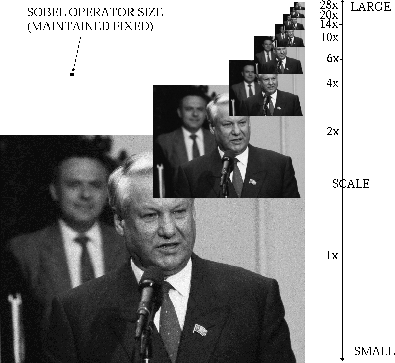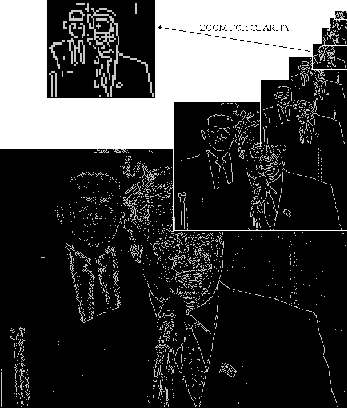To form the pyramid, a set of values of s are selected covering the desired scale space range. The ratio of s between adjacent scales (i.e., how finely we sample scale-space) depends on the flexibility of the subsequent operators with respect to scale. Figureillustrates the formation of an image pyramid via subaveraging and the corresponding sampling of scale space. Note, as well, that the Sobel operator that will be applied to the image pyramid is of fixed size. Figuredemonstrates the resulting multi-scale edge detection which is subsequently performed. Note the labelling at the side of the images which indicates large and small scales. The scale indicates the relative size of the operators, not the image. Thus, the smaller the intensity image, the larger the relative size of the operators acting upon it. The image at a scale value of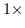is analyzed at a small scale since the operators are small relative to it and will detect tiny, local features in the image.

Thus, instead of resizing operators acting on the input image, the input is scaled and the operators are kept to a fixed size. This permits the use of simpler, faster, non-scalable operators such as the Sobel operator. Such a process is also more straightforward than painstakingly resizing a set of operators.

Scaling input images is also computationally efficient. For example, convolution with a mask of size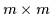with an image of sizerequires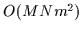operations. However, if the image and the operator are scaled by s, the convolution would require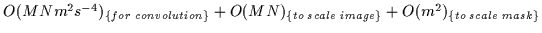. Large operators can thus be reduced to encourage computational efficiency as long as the input image is scaled appropriately.

Note, however, that the reduction in resolution brought about by scaling the image and the corresponding reduction in operator size gradually reduces the quality of the computation. Thus, it is necessary to select an operator size which acts on enough image pixels to perform the computation reliably.Next: Real-Time Symmetry Transform Up: Perceptual Contrast, Symmetry and Previous: Edge Data Enhancement
Tony Jebara
2000-06-23